# How to Calculate and Solve for Sedimentation of Concentrated Suspension | Rheology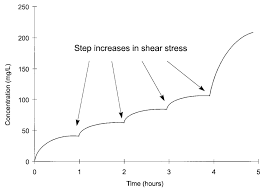The image above represents sedimentation of concentrated suspension.

To compute for sedimentation of concentrated suspension, five essential parameters are needed and these parameters are Change in Density between the Dispersed and Continuous Phase of the Suspension (ΔP), Acceleration due to Gravity (g), Particle Radius (a), Viscosity of Continuous Phase (η) and Solid Landing (φ).

The formula for calculating sedimentation of concentrated suspension:

v = 2ΔPga2 / (1 – φ)5 ± 0.25

Where:

v = Sedimentation of Concentrated Suspension
ΔP = Change in Density between the Dispersed and Continuous Phase of the Suspension
g = Acceleration due to Gravity
η = Viscosity of Continuous Phase
φ = Solid Landing

Let’s solve an example;
Find the sedimentation of concentrated suspension when the change in density between the dispersed and continuous phase of the suspension is 20, the acceleration due to gravity is 12, the particle radius is 32, the viscosity of continuous phase is 14 and the solid landing is 0.

This implies that;

ΔP = Change in Density between the Dispersed and Continuous Phase of the Suspension = 20
g = Acceleration due to Gravity = 12
a = Particle Radius = 32
η = Viscosity of Continuous Phase = 14
φ = Solid Landing = 0

v = 2ΔPga2 / (1 – φ)5 ± 0.25
v = 2 x 20 x 12 x 322 / 9 x 14 x (1 – 0)5 + 0.25
v = 2 x 20 x 12 x 1024 / 126 x (1)5.25
v = 491520 / 126 x 1
v = 3900.95 x 1
v = 3900.95

Therefore, the sedimentation of concentrated suspension is 3900.95.

Nickzom Calculator – The Calculator Encyclopedia is capable of calculating the sedimentation of concentrated suspension.

To get the answer and workings of the sedimentation of concentrated suspension using the Nickzom Calculator – The Calculator Encyclopedia. First, you need to obtain the app.

You can get this app via any of these means:

To get access to the professional version via web, you need to register and subscribe for NGN 2,000 per annum to have utter access to all functionalities.
You can also try the demo version via https://www.nickzom.org/calculator

Apple (Paid) – https://itunes.apple.com/us/app/nickzom-calculator/id1331162702?mt=8
Once, you have obtained the calculator encyclopedia app, proceed to the Calculator Map, then click on Materials and Metallurgical under Engineering.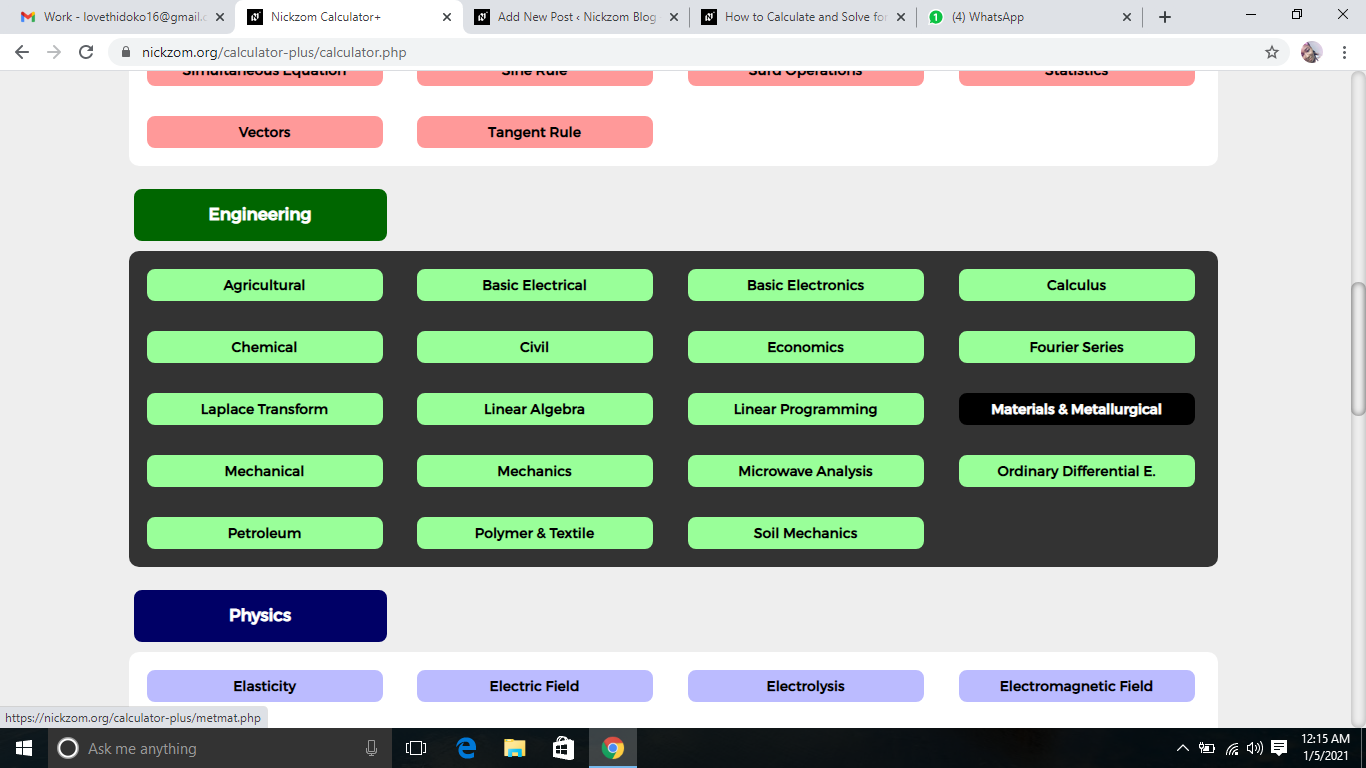Now, Click on Rheology under Materials and Metallurgical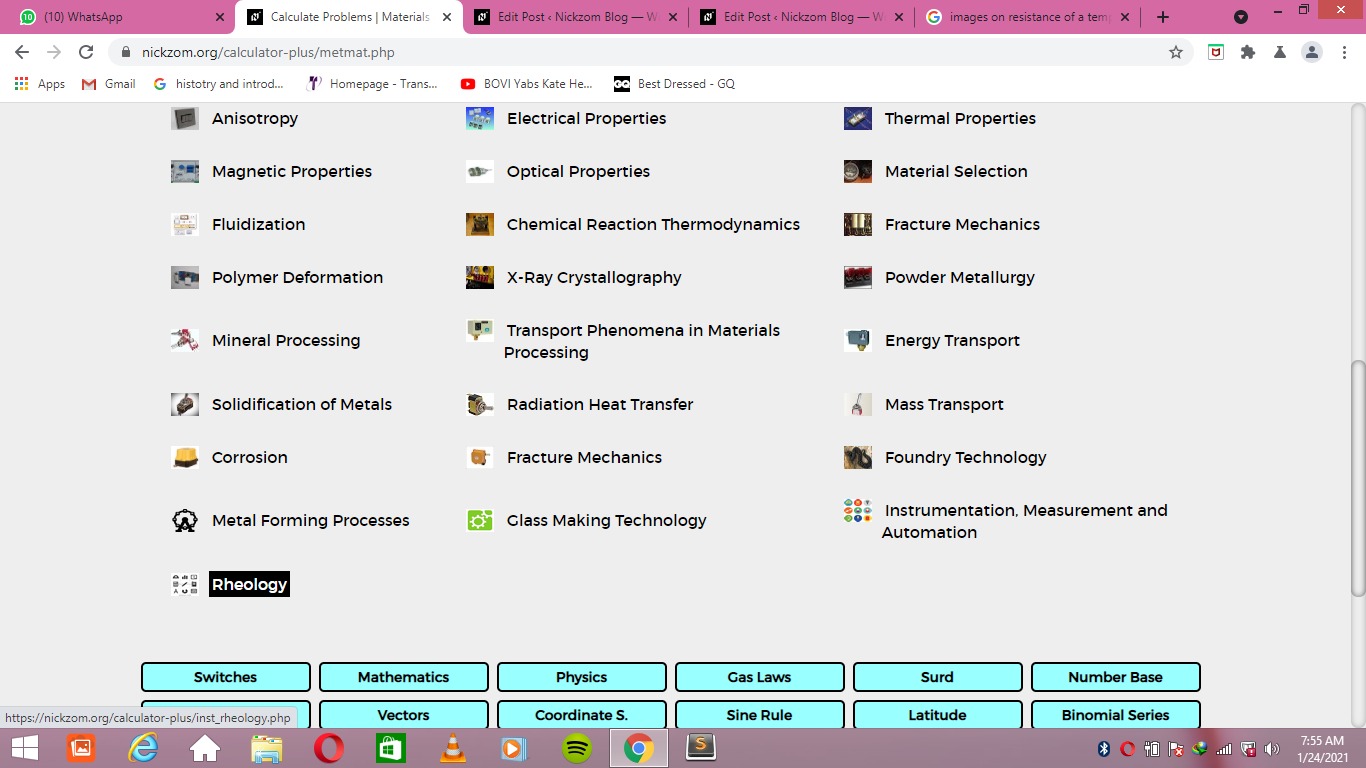Now, Click on Sedimentation of Concentrated Suspension under Rheology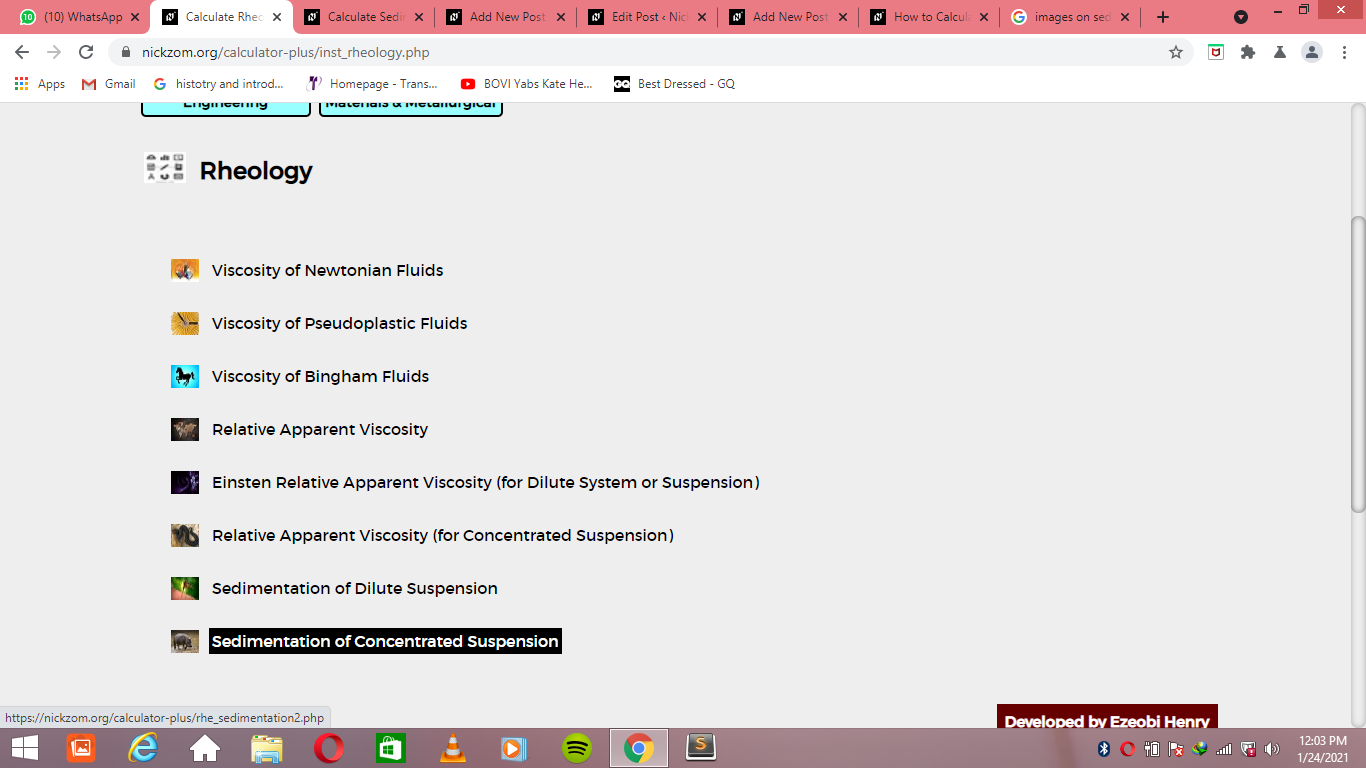The screenshot below displays the page or activity to enter your values, to get the answer for the sedimentation of concentrated suspension according to the respective parameters which is the Change in Density between the Dispersed and Continuous Phase of the Suspension (ΔP), Acceleration due to Gravity (g), Particle Radius (a), Viscosity of Continuous Phase (η) and Solid Landing (φ).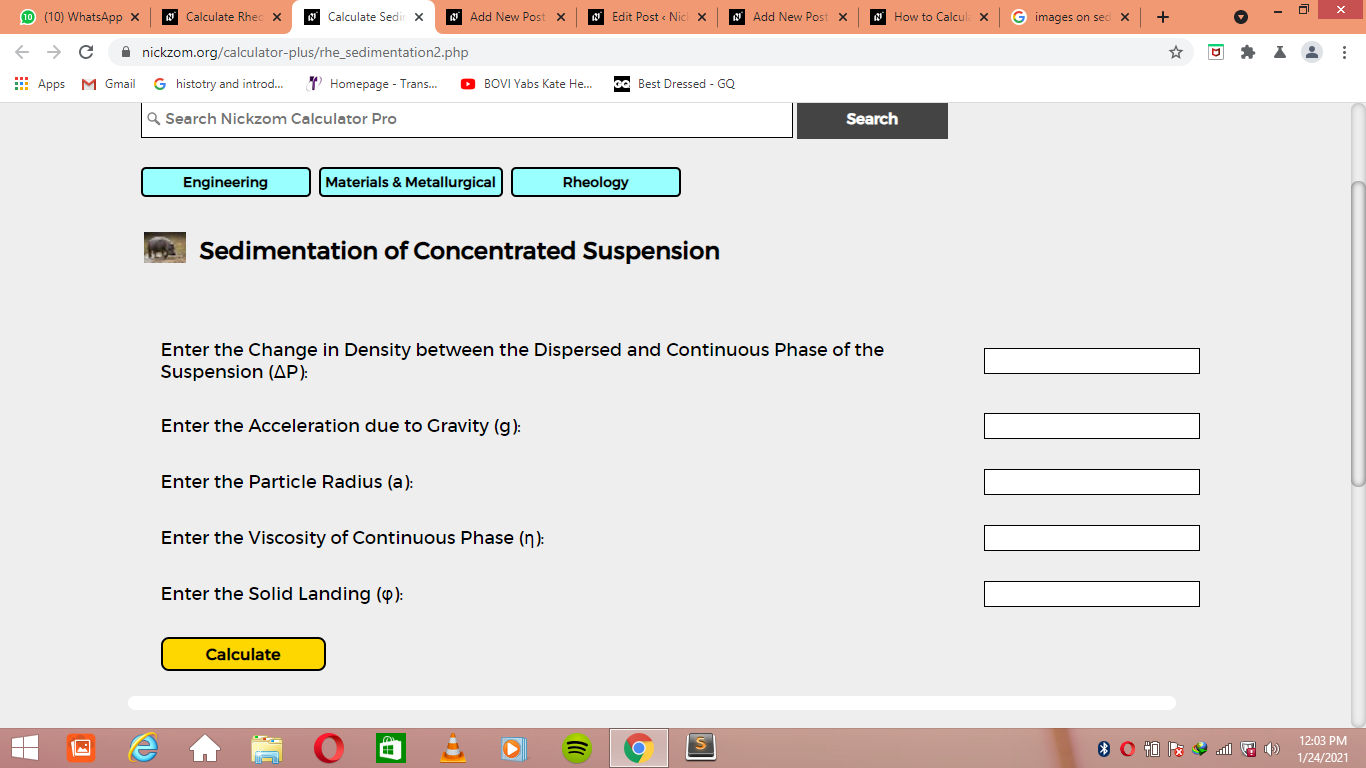Now, enter the values appropriately and accordingly for the parameters as required by the Change in Density between the Dispersed and Continuous Phase of the Suspension (ΔP) is 20, Acceleration due to Gravity (g) is 12, Particle Radius (a) is 32, Viscosity of Continuous Phase (η) is 14 and Solid Landing (φ) is 0.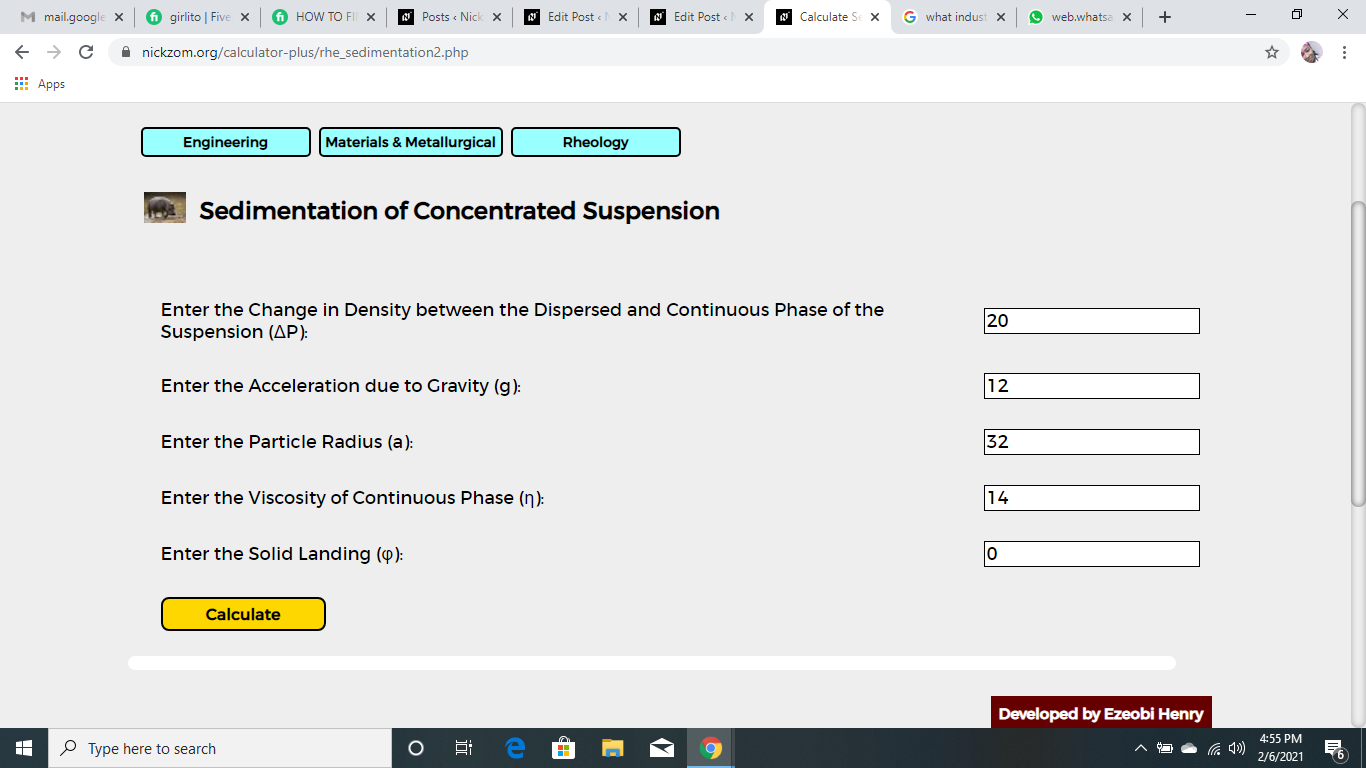Finally, Click on Calculate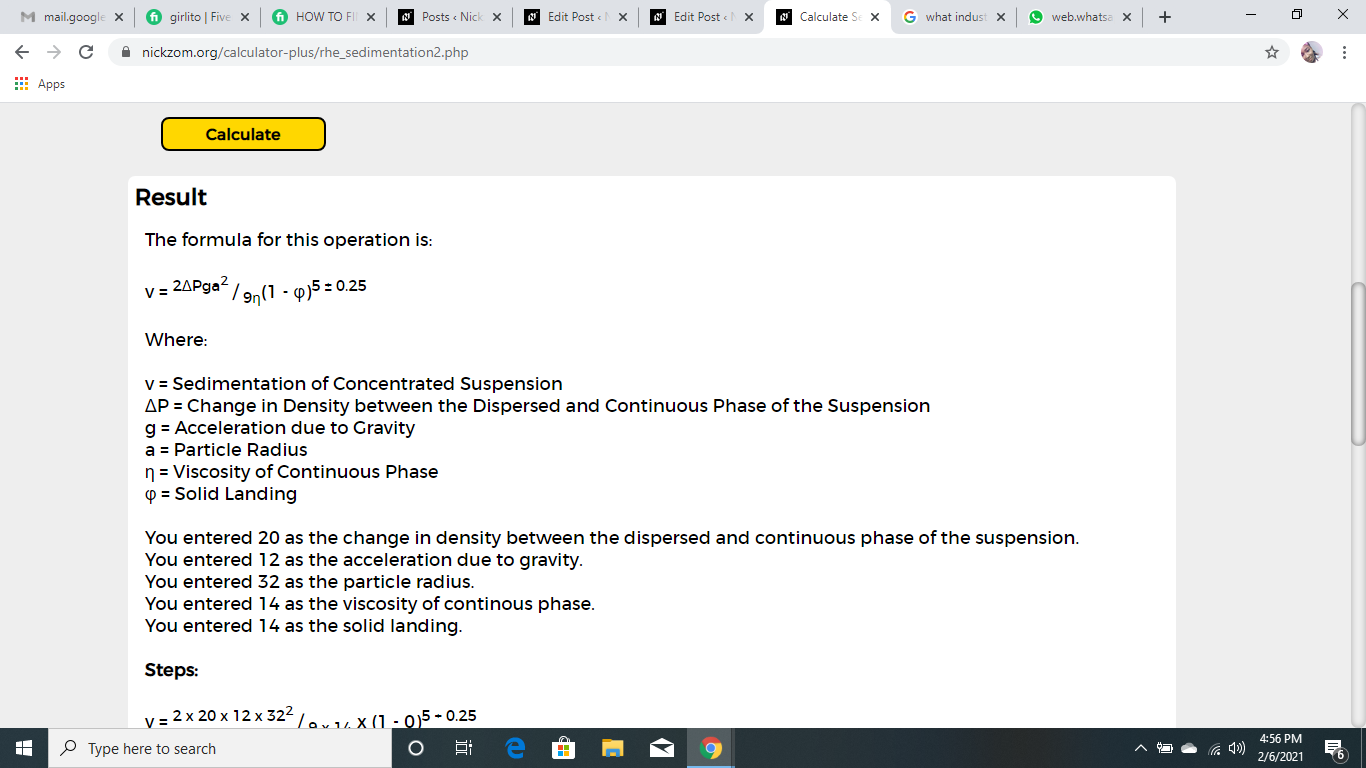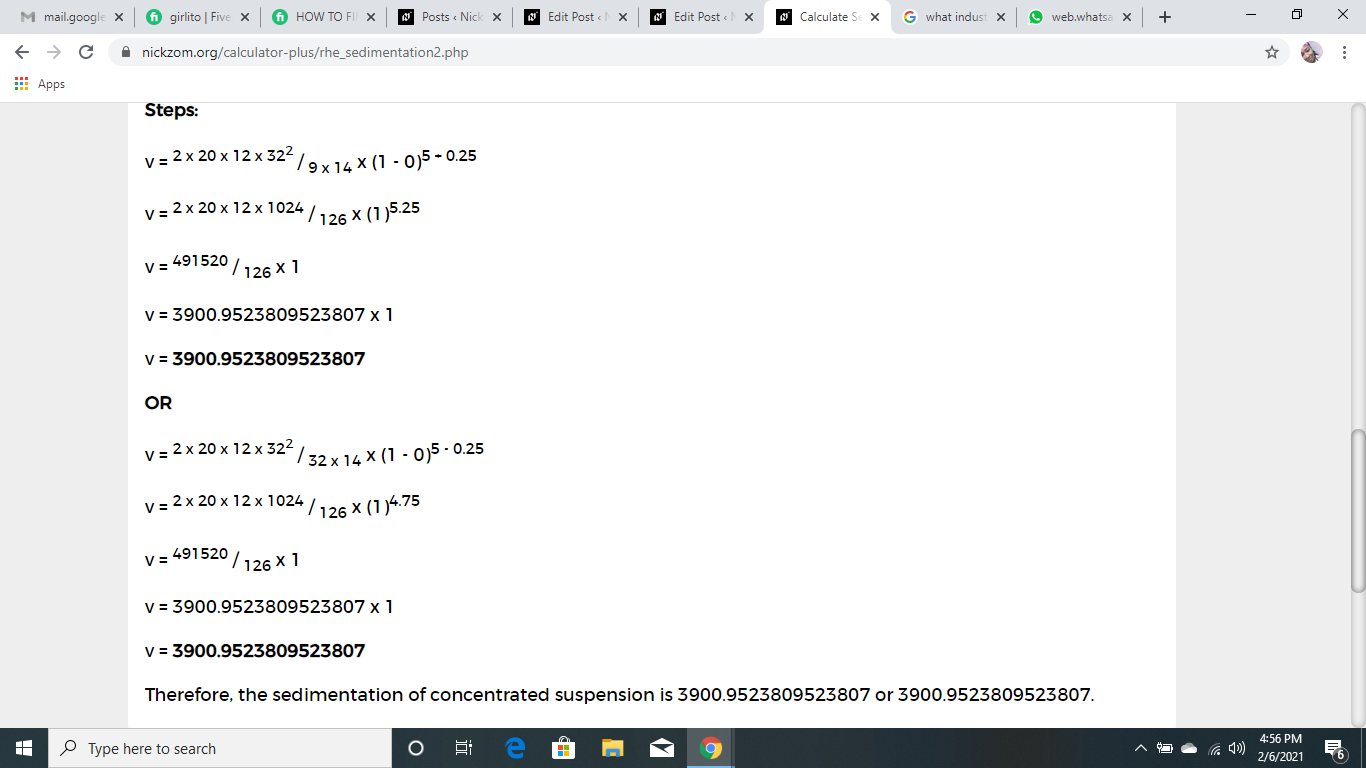As you can see from the screenshot above, Nickzom Calculator– The Calculator Encyclopedia solves for the sedimentation of concentration suspension and presents the formula, workings and steps too.